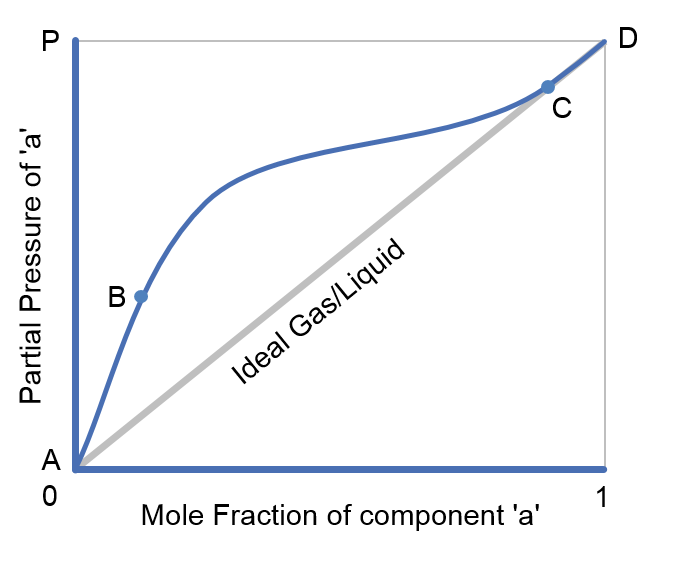f
Neutrium

# Gas

Henry's LawHenry’s Law describes the relationship between the solubility of a gas in a liquid and the partial pressure of that gas above the liquid surface. A range of experimentally determined Henry’s constants are tabulated and can be used to determine the solubility of various gas species in water.

Raoult's LawRaoult’s law gives a method of estimating the composition and pressure of the vapour above a liquid mixture. This article describes the basis of Raoult’s law and provides an example of how to apply it.

Dalton's Law

Dalton’s law provides a method by which the total pressure of a gas mixture can be calculated using the partial pressures of the component gases of the mixture.

Ideal Gas Law

The ideal gas law is used to relate volume, pressure and temperature for gases. The ideal gas law is an approximation and is generally more accurate at mild temperatures and pressures. The properties of gases increasingly deviate from ideal as conditions become more extreme, i.e. low temperature or high pressure. In these cases a compressibility factor may be used to correct for non-ideal behaviour when using the ideal gas relationship.

Conversion of Standard Volumetric Flow Rates of Gas

Standard volumetric flow rates of a fluid are the equivalent of actual volumetric flow rates in the sense that they have an equal mass flow rate. This identity makes standard volumetric flow appropriate providing a common baseline for comparison of volumetric gas flow rate measurements at different conditions. This article outlines how to convert between standard and actual volumetric flow rates.